Updated date:

# Parabola Equations and Graphs, Directrix and Focus and How to Find Roots of Quadratic Equations

Eugene is a qualified control/instrumentation engineer Bsc (Eng) and has worked as a developer of electronics & software for SCADA systems.

## The Parabola, a Mathematical Function

In this tutorial you'll learn about a mathematical function called the parabola. We'll cover the definition of the parabola first and how it relates to the solid shape called the cone. Next we'll explore different ways in which the equation of a parabola can be expressed. Also covered will be how to work out the maxima and minima of a parabola and how to find the intersection with the x and y axes. Finally we'll discover what a quadratic equation is and how you can solve it.

## Definition of a Parabola

"A locus is a curve or other figure formed by all the points satisfying a particular equation."

One way we can define a parabola is that it is the locus of points that are equidistant from both a line called the directrix and a point called the focus. So each point P on the parabola is the same distance from the focus as it is from the directrix as you can see in the animation below.

We notice also that when x is 0, the distance from P to the vertex equals the distance from the vertex to the directrix. So the focus and directrix are equidistant from the vertex.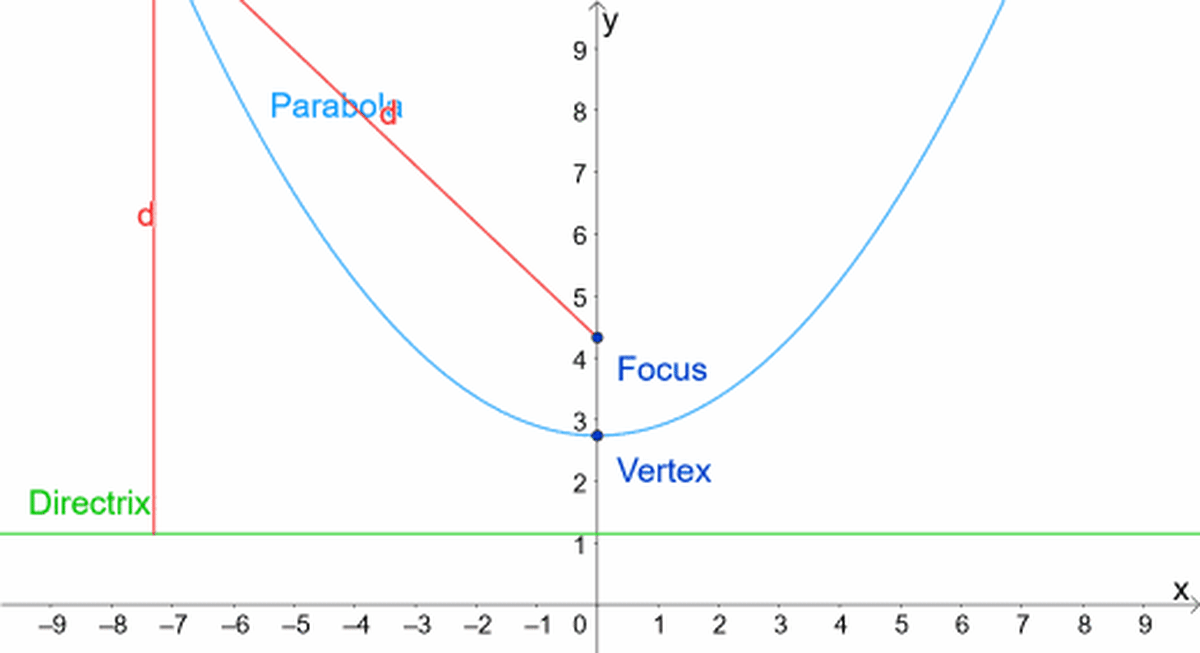A parabola is a locus of points equidistant (the same distance) from a line called the directrix and point called the focus.© Eugene Brennan

## A Parabola is a Conic Section

Another way of defining a parabola

When a plane intersects a cone, we get different shapes or conic sections where the plane intersects the outer surface of the cone. If the plane is parallel to the bottom of the cone, we just get a circle. As the angle A in the animation below changes, it eventually becomes equal to B and the conic section is a parabola.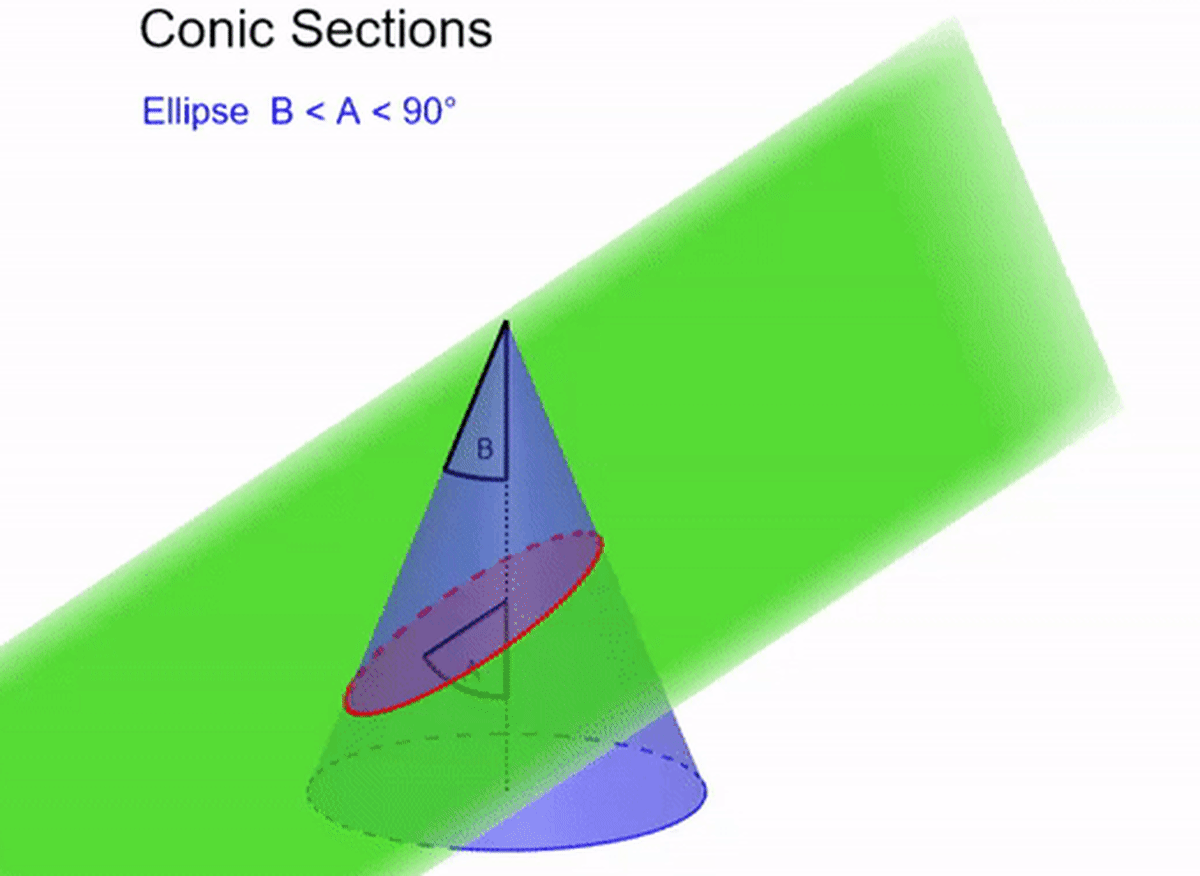A parabola is the shape produced when a plane intersects a cone and the angle of intersection to the axis is equal to half the opening angle of the cone.© Eugene Brennan

## Equations of Parabolas

There are several ways we can express the equation of a parabola:

• Vertex form
• Focus form

We'll explore these later, but first let's look at the simplest parabola.

## The Simplest Parabola y = x²

The simplest parabola with the vertex at the origin, point (0,0) on the graph, has the equation y = x².

The value of y is simply the value of x multiplied by itself.

xy = x²

1

1

2

4

3

9

4

16

5

25

## Let's Give x a Coefficient!

The simplest parabola is y = x2 but if we give x a coefficient, we can generate an infinite number of parabolas with different "widths" depending on the value of the coefficient ɑ.

So lets make y = ɑx2

In the graph below, ɑ has various values. Notice that when ɑ is negative, the parabola is "upside down". We'll discover more about this later. Remember the y = ɑx2 form of the equation of a parabola is when its vertex is at the origin.

Making ɑ smaller results in a "wider" parabola. If we make ɑ bigger, the parabola gets narrower.

## Turning the Simplest Parabola on Its Side

If we turn the parabola y = x2 on its side, we get a new function y2 = x or x = y2. This just means we can think of y as being the independent variable and squaring it gives us the corresponding value for x.

So:

When y = 2, x = y2 = 4

when y = 3, x = y2 = 9

when y = 4, x = y2 = 16

and so on...

Just like the case of the vertical parabola, we can again add a coefficient to y2.

So we have x = ɑy2

## Vertex Form of a Parabola Parallel to Y Axis

One way we can express the equation of a parabola is in terms of the coordinates of the vertex. The equation depends on whether the axis of the parabola is parallel to the x or y axis, but in both cases, the vertex is located at the coordinates (h,k). In the equations, ɑ is a coefficient and can have any value.

When the axis is parallel to y axis:

y = ɑ(x - h)2 + k

if ɑ = 1 and (h,k) is the origin (0,0) we get the simple parabola we saw at the start of the tutorial:

y = 1(x - 0)2 + 0 = x2

When the axis is parallel to the x axis:

x = ɑ(y - h)2 + k

Notice that this doesn't give us any information about the location of the focus or directrix.

## Equation of a Parabola in Terms of the Coordinates of the Focus

Another way of expressing the equation of a parabola is in terms of the coordinates of the vertex (h,k) and the focus.

We saw that:

y = ɑ(x - h)2 + k

Using Pythagoras's Theorem we can prove that the coefficient ɑ = 1/4p, where p is the distance from the focus to the vertex.

When the axis of symmetry is parallel to y axis:

Substituting for ɑ = 1/4p gives us:

y = ɑ(x - h)2 + k = 1/(4p)(x - h)2 + k

Multiply both sides of the equation by 4p:

4py = (x - h)2 + 4pk

Rearrange:

4p(y - k) = (x - h)2

or

(x - h)2 = 4p(y - k)

Similarly:

When the axis of symmetry is parallel to x axis:

A similar derivation gives us:

(y - k)2 = 4p(x - h)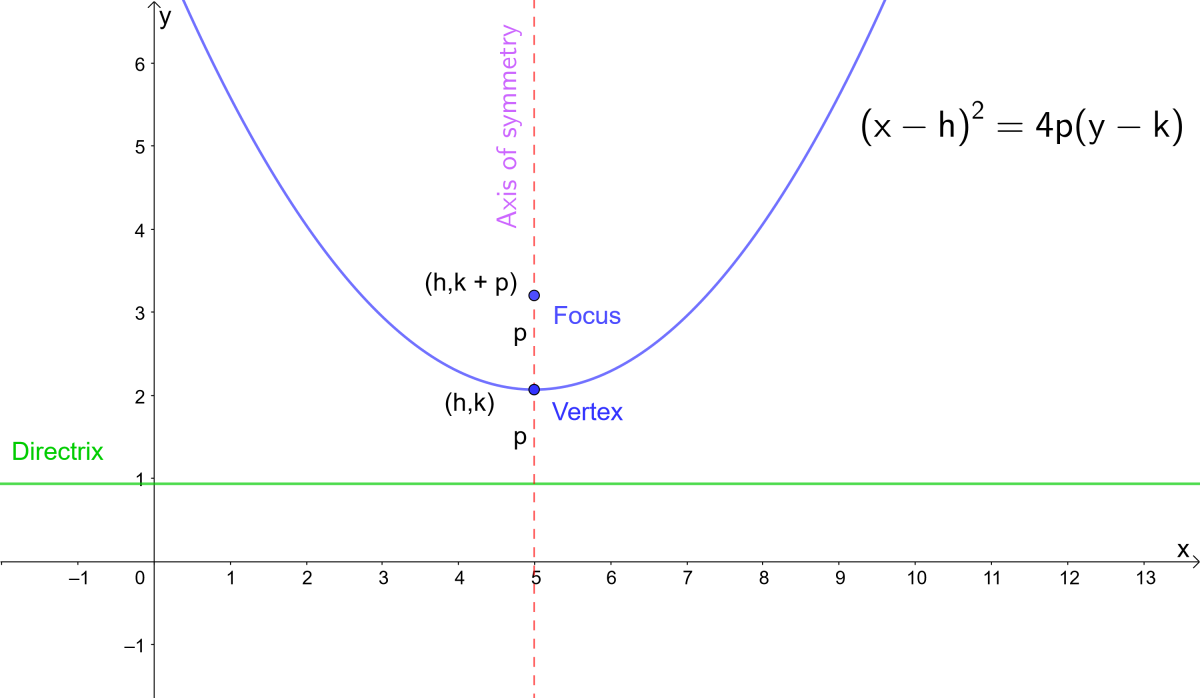Equation of a parabola in terms of the focus. p is the distance from the vertex to the focus and vertex to the directrix.© Eugene Brennan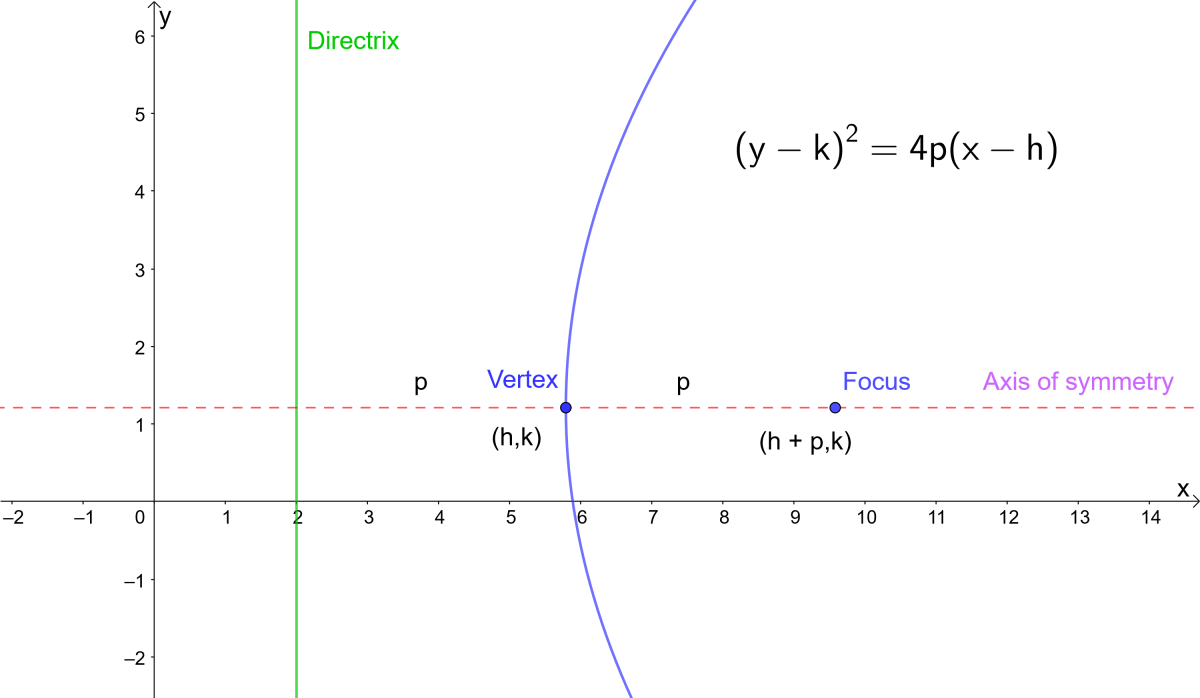Focus form of the equation of a parabola. p is the distance from the vertex to the focus and vertex to the directrix.© Eugene Brennan

Example:

Find the focus for the simplest parabola y = x2

Since the parabola is parallel to the y axis, we use the equation we learned about above

(x - h)2 = 4p(y - k)

First find the vertex, the point where the parabola intersects the y axis (for this simple parabola, we know the vertex occurs at x = 0)

So set x = 0, giving y = x2 = 02 = 0

and therefore the vertex occurs at (0,0)

But the vertex is (h,k), therefore h = 0 and k = 0

Substituting for the values of h and k, the equation (x - h)2 = 4p(y - k) simplifies to

(x - 0)2 = 4p(y - 0)

giving us

x2 = 4py

Now compare this to our original equation for the parabola y = x2

We can rewrite this as x2 = y, but the coefficient of y is 1, so 4p must equal 1 and p = 1/4.

From the graph above, we know the coordinates of the focus are (h, k + p), so substituting the values we worked out for h, k and p gives us the coordinates of the vertex as

(0, 0 + 1/4) or (0, 1/4)

## A Quadratic Function is a Parabola

Consider the function y = ɑx2 + bx + c

This is called a quadratic function because of the square on the x variable.

This is another way we can express the equation of a parabola.

## How to Determine Which Direction a Parabola Opens

Irrespective of which form of equation that is used to describe a parabola, the coefficient of x2 determines whether a parabola will "open up" or "open down". Open up means that the parabola will have a minimum and the value of y will increase on both sides of the minimum. Open down means it will have a maximum and the value of y decreases on both sides of the max.

• If ɑ is positive, the parabola will open up
• If ɑ is negative the parabola will open down

## Parabola Opens Up or Opens Down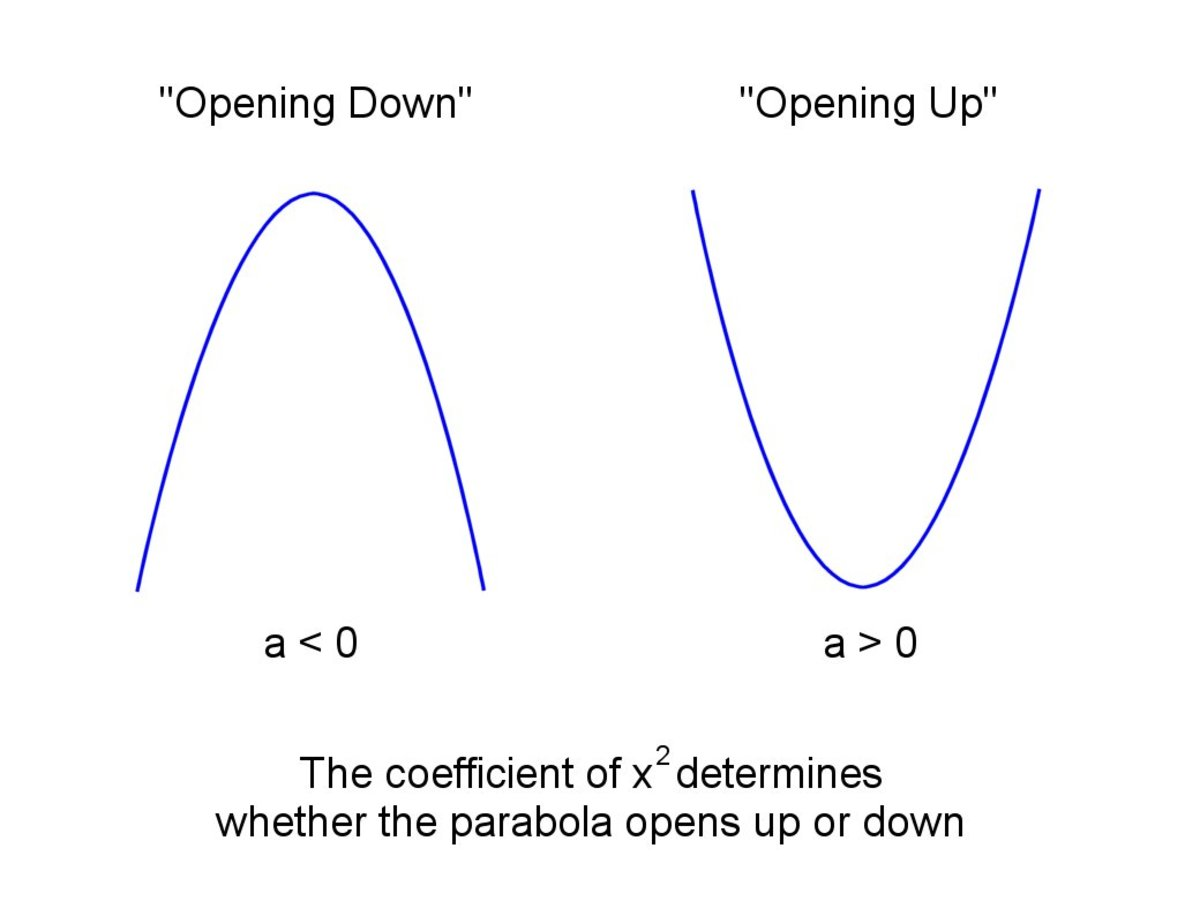The sign of the coefficient of x² determines whether a parabola opens up or opens down.© Eugene Brennan

## How to Find the Vertex of a Parabola

From simple calculus we can deduce that the max or min value of a parabola occurs at x = -b/2ɑ

Substitute for x into the equation y = ɑx2 + bx + c to get the corresponding y value

So y = ɑx2 + bx + c

= ɑ(-b/2ɑ)2 + b(-b/2ɑ) + c

= ɑ(b2/4ɑ2) - b2/2ɑ + c

Collecting up the b2 terms and rearranging

= b2 (1/4ɑ - 1/2ɑ) + c

= - b2/4ɑ + c

= c -b2/4a

So finally the min occurs at (-b/2ɑ, c -b2/4ɑ)

Example:

Find the vertex of the equation y = 5x2 - 10x + 7

1. The coefficient a is positive, so the parabola opens up and the vertex is a minimum
2. ɑ = 5, b = -10 and c = 7, so the x value of the minimum occurs at x = -b/2ɑ = - (-10)/(2(5)) = 1
3. The y value of the min occurs at c - b2/4a. Substituting for a, b and c gives us y = 7 - (-10)2 / (4(5)) = 7 - 100/20 = 7 - 5 = 2

So the vertex occurs at (1,2)

## How to Find the X-Intercepts of a Parabola

A quadratic function y = ɑx2 + bx + c is the equation of a parabola.

If we set the quadratic function to zero, we get a quadratic equation

i.e. ɑx2 + bx + c = 0 .

Graphically, equating the function to zero means setting a condition of the function such that the y value is 0, in other words, where the parabola intercepts the x axis.

The solutions of the quadratic equation allow us to find these two points. If there are no real number solutions, i.e. the solutions are imaginary numbers, the parabola doesn't intersect the x axis.

The solutions or roots of a quadratic equation are given by the equation:

x = -b ± √(b2 -4ac) / 2ɑ

## Finding the Roots of a Quadratic Equation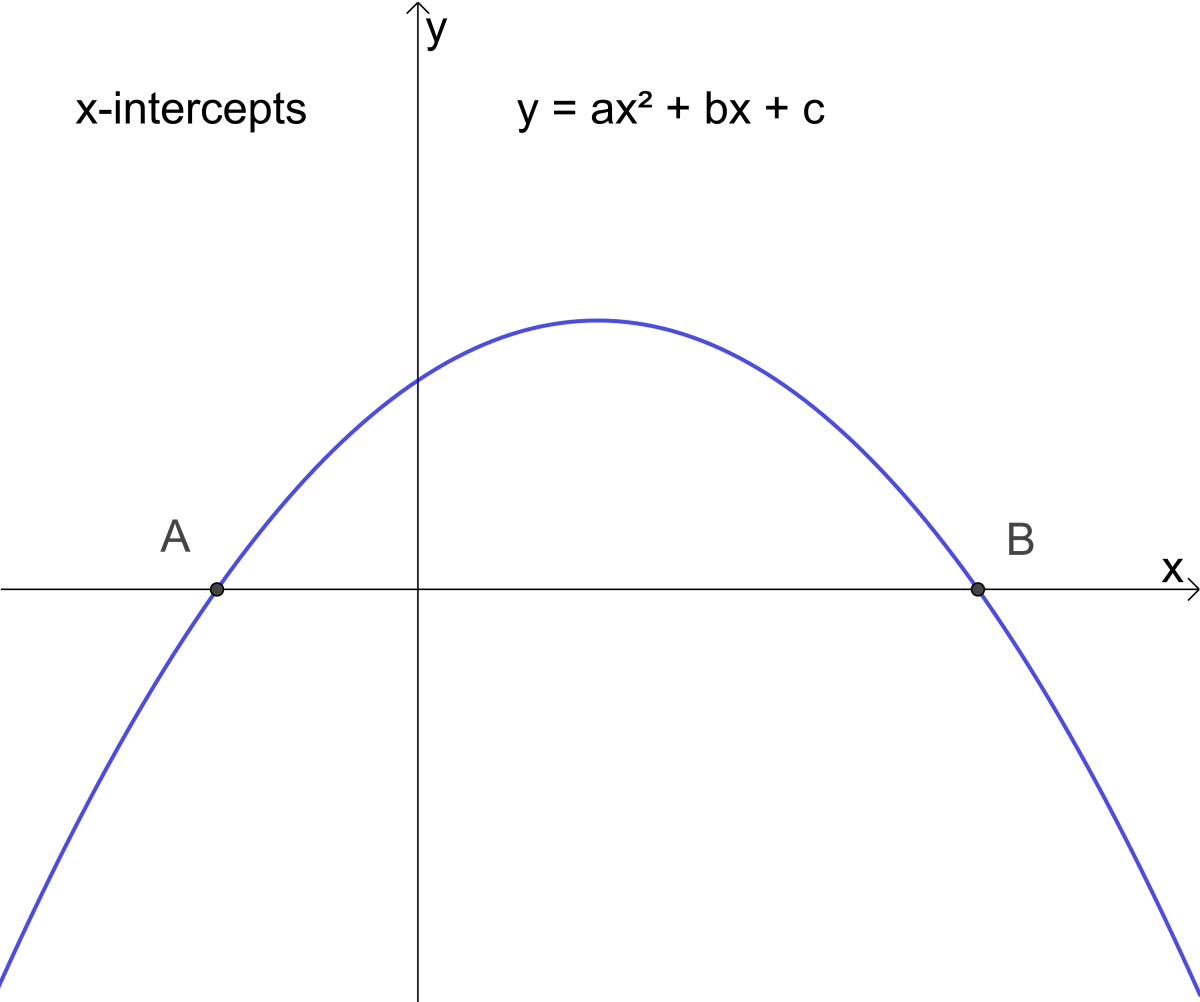A and B are the x-intercepts of the parabola y = ax² + bx + c and roots of the quadratic equation ax² + bx + c = 0© Eugene Brennan

Example 1: Find the x-axis intercepts of the parabola y = 3x2 + 7x + 2

Solution

• y = ɑx2 + bx + c
• In our example y = 3x2 + 7x + 2
• Identify the coefficients and constant c
• So ɑ = 3, b = 7 and c = 2
• The roots of the quadratic equation 3x2 + 7x + 2 = 0 are at x = (-b ± √(b2 - 4ɑc)) / 2ɑ
• Substitute for ɑ, b and c
• The first root is at x = (-7 + √(72 - 4 x 3 x 2)) / (2 x 3) = -1/3
• The second root is at (-7 - √(72 - 4 x 3 x 2)) / (2 x 3) = -2
• So the x axis intercepts occur at (-2, 0) and (-1/3, 0)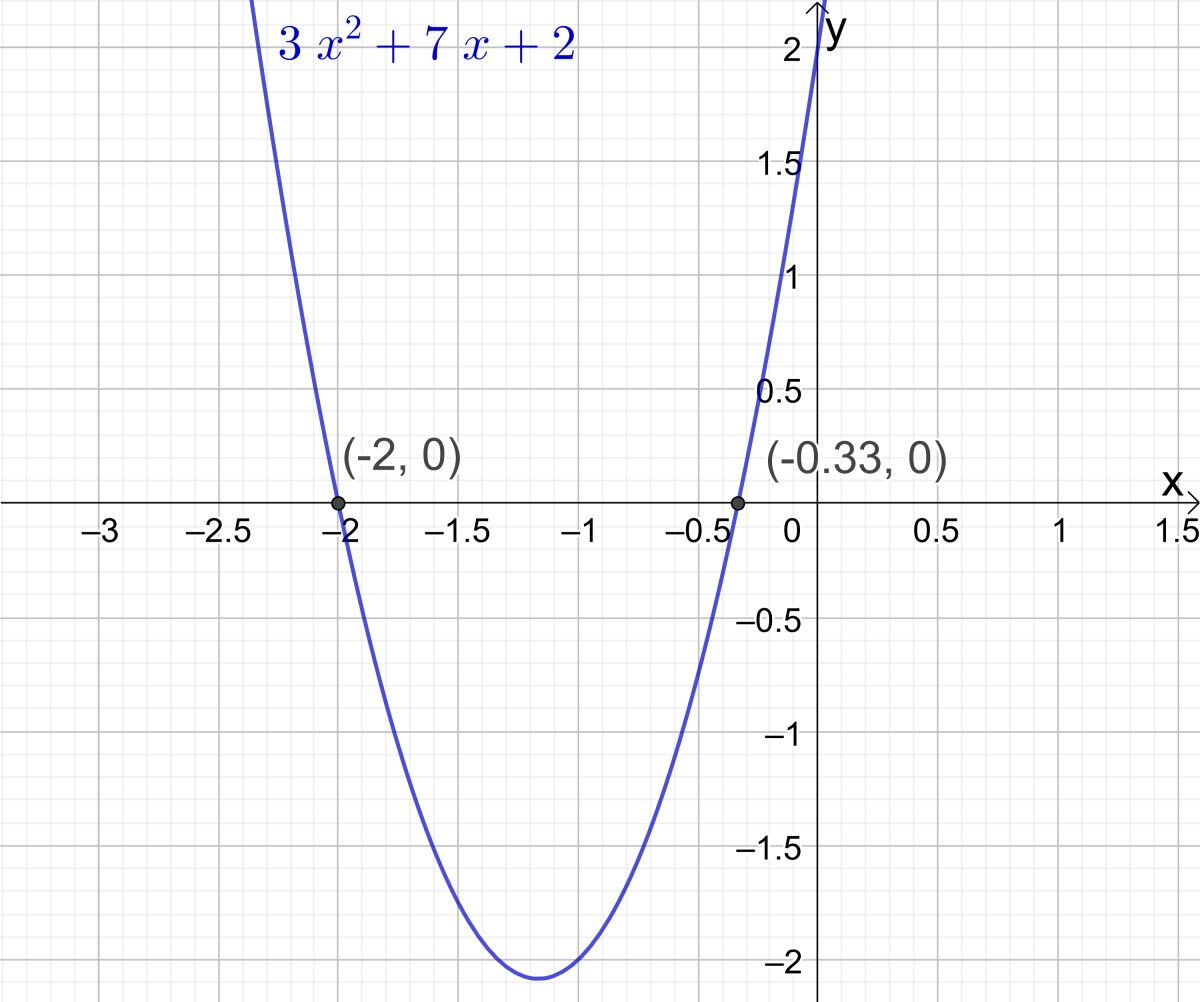Example 1: Find the x-intercepts of the parabola y = 3x2 + 7x + 2© Eugene Brennan

Example 2: Find the x-axis intercepts of the parabola with vertex located at (4, 6) and focus at (4, 3)

Solution

• The equation of the parabola in focus vertex form is (x - h)2 = 4p(y - k)
• The vertex is at (h,k) giving us h = 4, k = 6
• The focus is located at (h, k + p). In this example the focus is at (4, 3) so k + p = 3. But k = 6 so p = 3 - 6 = -3
• Plug the values into the equation (x - h)2 = 4p(y - k) so (x - 4)2 = 4(-3)(y - 6)
• Simplify giving (x - 4)2 = -12(y - 6)
• Expand out the equation gives us x2 - 8x + 16 = -12y + 72
• Rearrange 12y = -x2 + 8x + 56
• Giving y = -1/12x2 + 2/3x + 14/3
• The coefficients are a = -1/12, b = 2/3, c = 14/3
• The roots are at (-2/3 ± √((2/3)2 - 4(-1/12)(14/3)))/(2(-1/12)
• This gives us x = -4.49 approx and x = 12.49 approx
• So the x axis intercepts occur at (-4.49, 0) and (12.49, 0)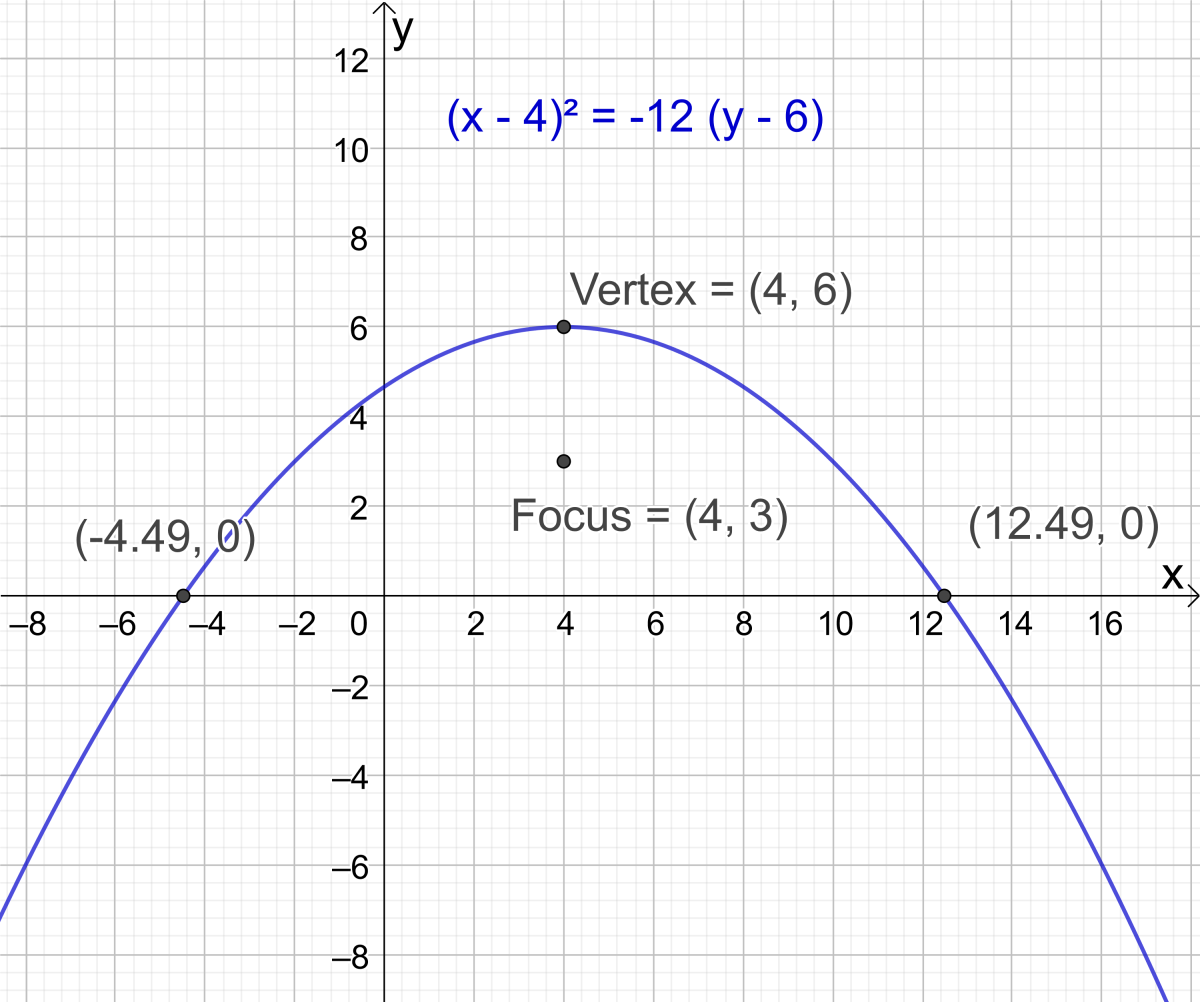Example 2: Find the x-intercepts of the parabola with vertex at (4, 6) and focus at (4, 3)© Eugene Brennan

## How to Find the Y-Intercepts of a Parabola

To find the y-axis intercept (y-intercept) of a parabola, we set x to 0 and calculate the value of y.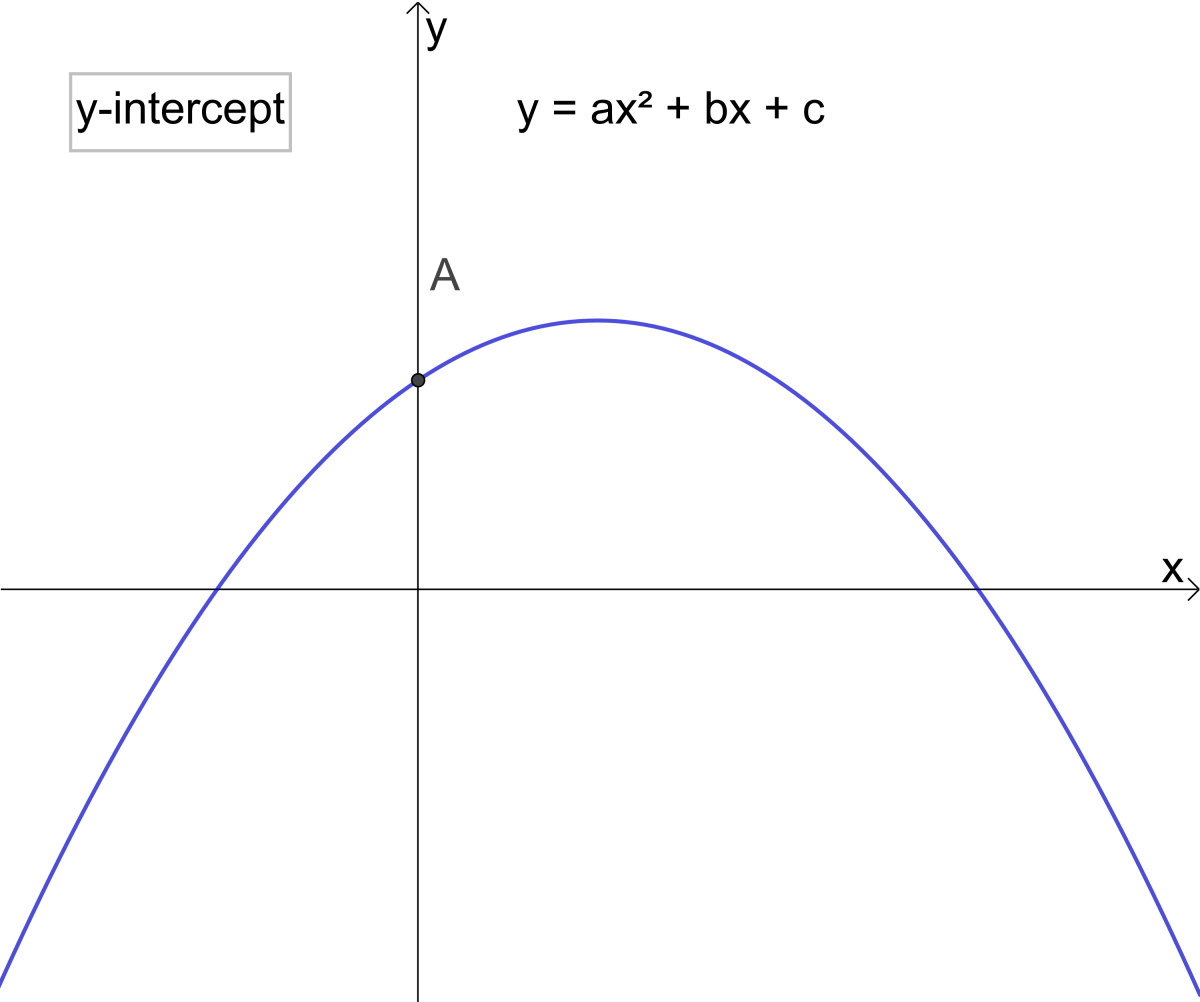A is the y-intercept of the parabola y = ax² + bx + c© Eugene Brennan

Example 3: Find the y-intercept of the parabola y = 6x2 + 4x + 7

Solution:

y = 6x2 + 4x + 7

Set x to 0 giving

y = 6(0)2 + 4(0) + 7 = 7

The intercept occurs at (0, 7)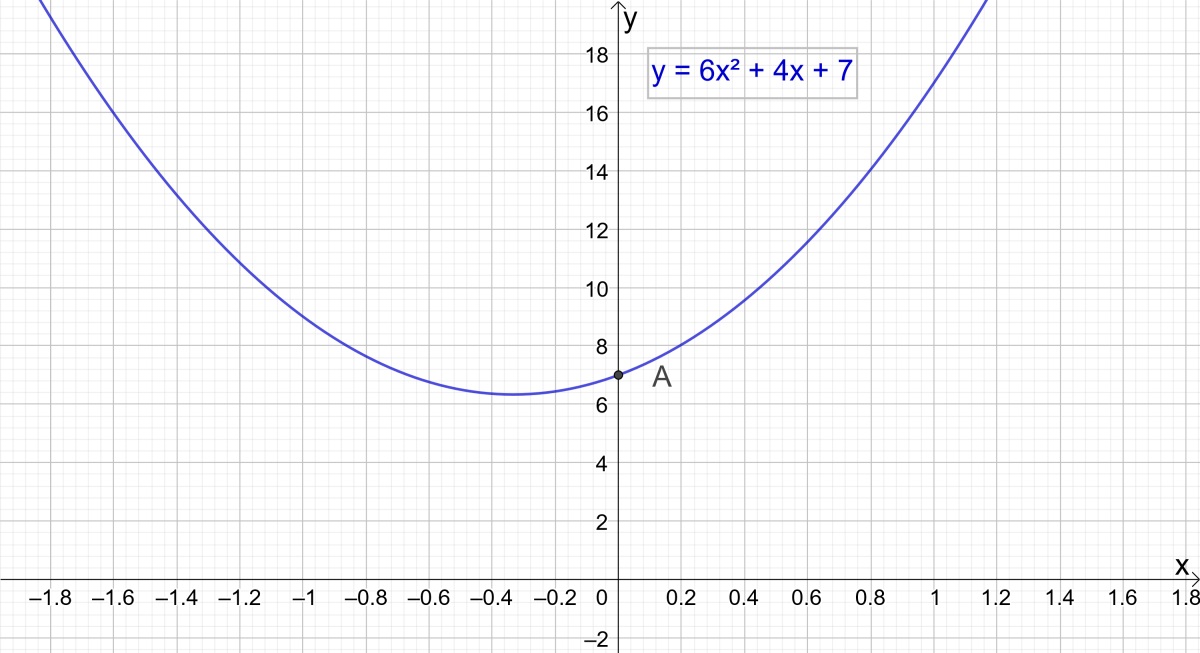Example 3: Find the y-intercept of the parabola y = 6x² + 4x + 7© Eugene Brennan

## Summary of Parabola Equations

For a parabola with axis parallel to the y-axis, (h,k) is the vertex and (h, k+ p) is the focus.

Equation TypeAxis Parallel to Y-AxisAxis Parallel to X-Axis

y = ɑx² + bx + c

x = ɑy² + by + c

Vertex Form

y = ɑ(x - h)² + k

x = ɑ(y - h)² + k

Focus Form

(x - h)² = 4p(y - k)

(y - k)² = 4p(x - h)

Parabola with Vertex at the Origin

x² = 4py

y² = 4px

Roots of a parabola parallel to y axis

x = -b ± √( b² -4ɑc)/2ɑ

Vertex occurs at

(-b/2ɑ, c -b2/4ɑ)

## How the Parabola is Used in the Real World

The parabola isn't just confined to math. The parabola shape appears in nature and we use it in science and technology because of its properties.

• When you kick a ball into the air or a projectile is fired, the trajectory is a parabola
• The reflectors of vehicle headlights or flashlights are parabolic shaped
• The mirror in a reflecting telescope is parabolic
• Satellite dishes are in the shape of a parabola as are radar dishes

For radar dishes, satellite dishes and radio telescopes, one of the parabola's properties is that a ray of electromagnetic radiation parallel to its axis will be reflected towards the focus. Conversely in the case of a headlight or torch, light coming from the focus will be reflected off the reflector and travel outwards in a parallel beam.

Related reading: Deriving Projectile Motion EquationsWater from a fountain (which can be considered as a stream of particles) follows a parabolic trajectoryGuidoB, CC by SA 3.0 Unported via Wikimedia Commons

## Acknowledgements

All graphics were created using GeoGebra Classic.